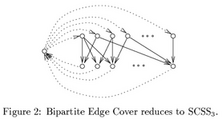## neal young / Khuller96Strongly

•The MEG (minimum equivalent graph) problem is, given a directed graph, to find a small subset of the edges that maintains all reachability relations between nodes. The problem is NP-hard; This paper gives a proof that, for graphs where each directed cycle has at most three edges, the MEG problem is equivalent to maximum bipartite matching, and therefore solvable in polynomial time. This leads to an improvement in the performance guarantee of the previously best approximation algorithm for the general problem, from Approximating the minimum equivalent digraph'' .

(This result was improved by Berman et al, WADS, 2009.)